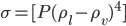Escobedo and Mansoori (AIChE J. 42(5): 1425, 1996) Found original paper: check Escobedo 1996

The proposed 1996 paper relates the surface tension of mixtures to bulk-phase concentrations and properties (i.e. densities). Surface tension of pure organic fluids σ:$\sigma=[P(\rho_l-\rho_v)^4]$

The two ρ are densities: liquid and vapour.

They introduced parachor (P), a new expression, derived from statistical mechanics (from Macleod equation) which represents the experimental surface tension of 94 different organic compounds within 1.05 AAD% (average absolute deviations).

(Kumar 2005) It expresses the surface tension of a liquid in equilibrium with its own vapour. Historical reference for parachor cited here: Macleod, Sugden, Quayle, Escobedo.